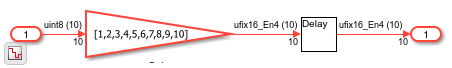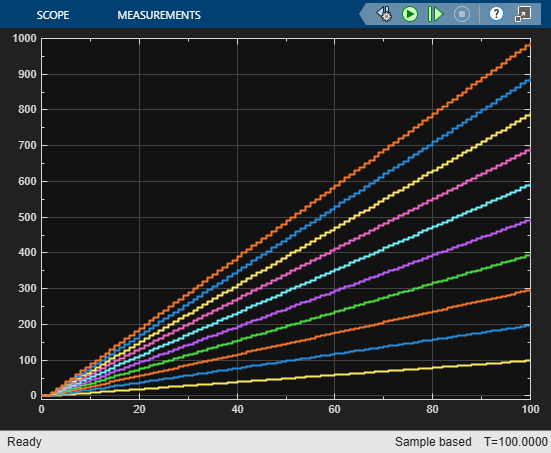## Resource Sharing Guidelines for Vector Processing and Matrix Multiplication

Resource sharing is an area optimization in which HDL Coder™ identifies multiple functionally equivalent resources and replaces them with a single resource. The data is time-multiplexed over the shared resource to perform the same operations. To learn more about how resource sharing works, see Resource Sharing.

You can follow these guidelines to learn how to use the resource sharing with streaming when processing 1-D vectors and 2-D matrices. Each guideline has a severity level that indicates the level of compliance requirements. To learn more, see HDL Modeling Guidelines Severity Levels.

### Use StreamingFactor for Resource Sharing of Vector Signals

Guideline ID

`3.1.9`

Severity

Informative

Description

To reduce circuit area of a Subsystem block that performs the same computation on each element of a 1-D vector, use the Subsystem HDL block property StreamingFactor. For a vector signal that has N elements, set StreamingFactor to `N`. By using time-division multiplexing to process each element, you can process the result by using smaller number of operations. The clock frequency of the operators becomes N times faster than that of the original model.

When the subsystem containing resources to be shared uses multiple vector signals with different sizes, the clock frequency is multiplied by the least common multiple of the vector sizes, which can reduce the maximum achievable target frequency. To achieve the desired frequency:

• Add logic for demultiplexing the vector signal before it enters the subsystem and for multiplexing the signal that leaves the subsystem. You can then specify a SharingFactor on the subsystem instead of the StreamingFactor.

• Pad the different vector signals to make them the same size as the vector signal that has the maximum size, and then specify the StreamingFactor.

Open the model `hdlcoder_vector_stream_gain`.

```open_system('hdlcoder_vector_stream_gain') set_param('hdlcoder_vector_stream_gain', 'SimulationCommand', 'Update') ```The model accepts a 10-element vector signal as input and multiplies each element by a gain value that is one more than the previous value.

```open_system('hdlcoder_vector_stream_gain/Gain_Stream') ```To see the simulation results, simulate the model and open the Scope block.

```sim('hdlcoder_vector_stream_gain') open_system('hdlcoder_vector_stream_gain/Show Processing Time') ```The `Gain_Stream` subsystem has a StreamingFactor set to `10`. To generate HDL code for this subsystem, run the `makehdl` function:

```makehdl('hdlcoder_vector_stream_gain/Gain_Stream') ```

After generating HDL code, to see the effect of the streaming optimization, open the generated model and navigate inside the `Gain_Stream` subsystem.The vector data is serialized on the input side and the output size parallelizes the serial data. This optimization increases the total circuit size conversely when the target circuit size to be shared is small. The Gain block inside the shared subsystem is running at a rate that is 10 times faster than the model base rate, which avoids an increase in the subsystem latency and balances the reduction in maximum achievable frequency by the increase in area savings on the target hardware.

### Use SharingFactor and HDL Block Properties for Sharing Matrix Multiplication Operations

`3.1.10`

Informative

#### Description

The Matrix Multiply block is a Product block that has Multiplication block parameter set to `Matrix(*)`. In the HDL Block Properties dialog box, the HDL architecture is set to `Matrix Multiply` and you can specify the DotProductStrategy.

DotProductStrategy Settings

DotProductStrategyDescription
`'Fully Parallel'` (default)Performs multiplication and addition operations in parallel. `[MxN]*[NxM]` matrix multiplication requires `N*M*M` multipliers.
`'Parallel Multiply-Accumulate'`Uses the Parallel architecture of the Multiply-Accumulate block to implement the matrix multiplication. This architecture performs multiple Multiply-Add blocks in parallel with accumulation.
`'Serial Multiply-Accumulate'`Uses the Serial architecture of the Multiply-Accumulate block to implement the matrix multiplication. This mode performs `N` times oversampling and number of multipliers becomes `M*M`.

To share resources and reduce the number of multipliers further, when you have multiple Matrix Multiply blocks in the same subsystem, set DotProductStrategy to ```Fully Parallel``` and specify the SharingFactor on the upper subsystem.

For multiplications involving complex and real numbers, the number of multipliers become doubled.

Number of Multipliers Generated by Multiplication of [MxN]*[NxM]

Multiplication TypeFully Parallel/Parallel Multiply-AccumulateSerial Multiply-Accumulate
Real x Real`N*M*M``M*M`
Complex x Real`N*M*M*2``M*M*2`
Complex x Complex`N*M*M*4``M*M*4`

For floating-point matrix multiplication, select Use Floating Point. In this case, you must use the `Fully Parallel` DotProductStrategy. As this mode does not use element-wise operations and performs parallel multiplication and addition operations, use the SharingFactor instead of the StreamingFactor to share resources and save circuit area.

For an example that shows how to perform streaming matrix multiplication using floating-point types, see HDL Code Generation for Streaming Matrix Multiply System Object.